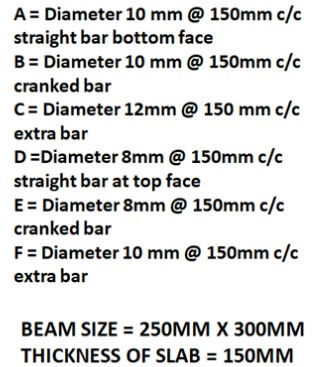top of page
Search

# Bar Bending Schedule (BBS) Calculation for Reinforcement in Slab Construction

To calculate the Bar Bending Schedule (BBS) for a slab, you need to consider various factors such as the dimensions of the slab, the reinforcement requirements, and the design specifications. Here's an example of how you can calculate the BBS for a slab:

In the structural Drawing, the Reinforcement detail of the Slab is given,NOTE: Length of an extra bar at continuous support of slab will be L/4 on both sides from the center of support where L is the span from center to center

For the bar bending schedule for the slab, Firstly we have to find out the cutting length of the bars in placed shorter span

Here A Bars are Straight bars of Diameter 10mm and spacing= 150mm c/c

B Bars are cranked bars of diameter 10mm and spacing = 150mm c/c

C Bars are extra bars of diameter 12mm and Spacing = 150mm c/c

Step 1:-Cutting Length of Bars

1. cutting length of A bars = 3000[-(25+25)] (subtracting 25mm beam covers both side)

=2950mm or 2.95m• Cutting length of B bars = 3000[-(25+25)] + 0.42t+0.42t

(Where T= thickness of slab, t = Thickness of slab – Top and Bottom Covers)

t = 150-(20+20) Covers top and bottom

t = 110mm

Therefore, 2.950+2x(0.42 x 0.11)

= 2.950+0.092=3.042m

• Cutting Length of C bars = C/C length

= 3-(0.125+0.125)

= 2.75m

According to drawing Cutting length of the extra bar is L/4

Therefore, 2.75/4 = 0.6875 + 0.1m

= 0.7875m

Step 2:- No of Bars

1. A Bars :- opposite length(-covers)/spacing

= 10000-(25+25)/150

=9950/150= 66.33nos.

• B Bars (no. of bars of cranked bars are 1 more than straight bars as they are placed alternatively).

Therefore, 66.33+1= 64.33 nos

• C Bars Extra bars= Same as Straight bars =63.33 nos

Step 3:- the unit weight of Bar

1. for 12mm bars= D²/162

= 12×12/162=0.888Kg/m

• for 10mm bars = D2/162

= 10 x 10/162

=0.617kg/m

Step 4:-Total Weight of Bars:

1. Total weight of A bars = cutting length × No. of Bars × unit weight

= 2.95m ×63.33 × 0.617

= 115.27 kg of Bars are used

• Total Weight of B bars= cutting length × No. of Bars × unit weight

= 3.042m ×64.33 × 0.617

= 120.74 kg of Bars are used

• Total Weight of C bars= cutting length × No. of Bars × unit weight

= 0.6875m ×63.33 × 0.888

= 38.66 kg of Bars are used

Now we have to find out the cutting length of the bars placed in longer span

Here D Bars are Straight bars of Diameter 10mm and spacing= 150mm c/c

E Bars are cranked bars of diameter 10mm and spacing = 150mm c/c

F Bars are extra bars of diameter 12mm and Spacing = 150mm c/c

Step 1:-

• cutting length of D bars = 10000[-(25+25)] (subtracting 25mm beam covers both side)

=9950mm or 9.95m• utting length of E bars = 10000[-(25+25)] + 0.42t + 0.42t + 042t + 0.42t

(Where T= thickness of slab, t = Thickness of slab – Top and Bottom Covers)

t = 150-(20+20) Covers top and bottom

t = 110mm

Therefore, 10+4x(0.42 x 0.11)

= 9.950+0.184=10.134m

• Cutting Length of F bars = C/C length

= 10-(0.125+0.125)

= 9.75m

According to drawing Cutting length of extra bar is L/4

Therefore, 9.75/4 = 2.4375m

Step 2:- No of Bars

• D Bars :- opposite length(-covers)/spacing

= 3000-(25+25)/150

=2950/150= 19.66nos.

• E Bars (no. of bars of cranked bars are 1 more than straight bars as they are placed alternatively).

Therefore, 19.66+1= 20.66 nos

• F Bars Extra bars= Same as Straight bars =19.66 nos

Step 3:- the unit weight of Bar

• for 12mm bars= D²/162

= 12×12/162=0.888Kg/m

• for 10mm bars = D2/162

= 10 x 10/162

=0.617kg/m

Step 4:-Total Weight of Bars:

• Total weight of D bars = cutting length × No. of Bars × unit weight

= 9.95m ×19.66 × 0.617

= 120.69 kg of Bars are used

• Total Weight of E bars= cutting length × No. of Bars × unit weight

= 10.134m ×20.66 × 0.617

= 129.18 kg of Bars are used

• Total Weight of F bars= cutting length × No. of Bars × unit weight

= 2.437m ×19.66 × 0.888

= 42.54kg of Bars are used